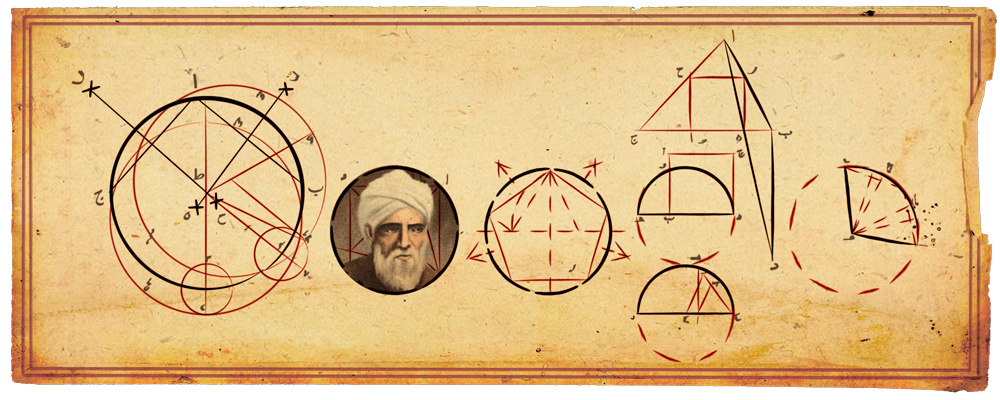# Abû al-Wafâ’ al-Buzjanî’s doodleThis beautiful doodle was published by google in the Persian and Arabic countries last 10th June because in 10th June 940 the great Abû al-Wafâ’ al-Buzjanî was born in Persia. Since 959, he worked in the Caliph’s court in Baghdad among other distinguished mathematicians and scientists who remained in the city after Sharâf al-Dawlâh became the new caliph in 983. He continued to support mathematics and astronomy and built a new observatory in the gardens of his palace in Baghdad (June 988) which included a quadrant over 6 metres long and a sextant of 18 metres.

Abû al-Wafâ’ wrote commentaries on works of Euclid, Ptolemy, Diophantus and al-Khwârizmî, and his works were very important in the developement of Trigonometry and Astronomy.

# Fermat’s theorem

Another of the important equations which stands out in the main entrance of Cosmocaixa in Barcelona is Fermat’s last theorem:

Fermat was reading Diophantus’ Arithmetic about the pythagorean triples x2 + y2 = z2 in 1637 when he noticed immediately that n = 2 was the only case which satisfies the equation xn + yn = zn. Then, he wrote in the margin of his edition of Diophantus’ work:

it is impossible to separate a cube into two cubes, or a fourth power into two fourth powers, or in general, any power higher than the second, into two like powers. I have discovered a truly marvelous proof of this, which this margin is too narrow to contain

Probably, Fermat was only able to prove the new theorem for n = 4 using his proof by infinite descent: Fermat proved that there are not three numbers x, y, z such that  x4 + y4 = z2 . First of all, we can suppose that x, y, z are co-prime from (x2)2 + (y2)2 = z2. If <x,y,z> = d, then we can divide all the equation by d2 and we’ll have another equation (x2)2 + (y2)2 = z2 with <x,y,z> = 1.

Now, from the solution of the Pythagorean triples, there are pq such that x2 = 2pq, y2 = p2 – q2 and z = p2 + q2.

We can observe that  y2 = p2 – q2 implies that p2 = q2 – q2 is another Pythagorean triple so there are uv co-prime such that q = 2uvy = u2 – v2 and p = u2 + v2.

So, x2 = 2pq = 2(u2 + v2)(2uv) = 4(uv)(u2 + v2). Since <p,q> = 1, we know that <uv,u2 + v2> = 1 and since their product by 4 is a perfect square, they must be perfect squares too. So there exists a < p such that a2u2 + vp.

Finally, if we had (x2)2 + (y2)2 = z2 , we have obtain p and q co-prime with  z = p2 + q2. Then it is possible to obtain another pair u and v co-prime with p u2 + vand p < z, u < p and v < q. So we can iterate this algorithmic procedure so we’ll obtain a set of pairs of natural numbers each of them lower than the previous and this fact is false, since we’d have an infinite decreasing succession of natural numbers without end!

Nobody accepts that Fermat could have known any other case apart of n = 4! In 1753, Leonhard Euler wrote a letter to Christian Goldbach telling him that he had proved the case n = 3 but the demonstration that he published in his Algebra (1770) was wrong. He tried to find integers p, q, z such that (p2 + 3q2) = zand he found that:

p = a3 – 9ab2q = 3(a2b – b3) ⇒ p2 + 3q2 = (a2 + 3b2)3

He worked with numbers of the form a + b√-3 to find two new numbers a and b less than p and q such that p2 + 3q2 = cube and then he applied the method of infinite descent. Unluckily, he made some mistakes working with the new complex numbers  a + b√-3.

This story is so exciting but, almost quoting Fermat, this post is too short to contain everything. If you are interested in, you must read Simon Singh’s Fermat last theorem which is as interesting as the theorem itself.